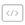• 创建数组
• 数学函数
• 数组索引
• 数组统计
• 数组运算
• 数组切片
• 数组排序实验 1 NumPy 百题大冲关｜学习版实验 2 NumPy 百题大冲关｜题目版

## 实验任务

• 使用 NumPy 创建数组
• 使用 NumPy 对数组进行运算
• 使用 NumPy 对数组进行排序
• 使用 NumPy 对数组形状进行操作
• 使用 NumPy 对数组进行统计

## 面向用户